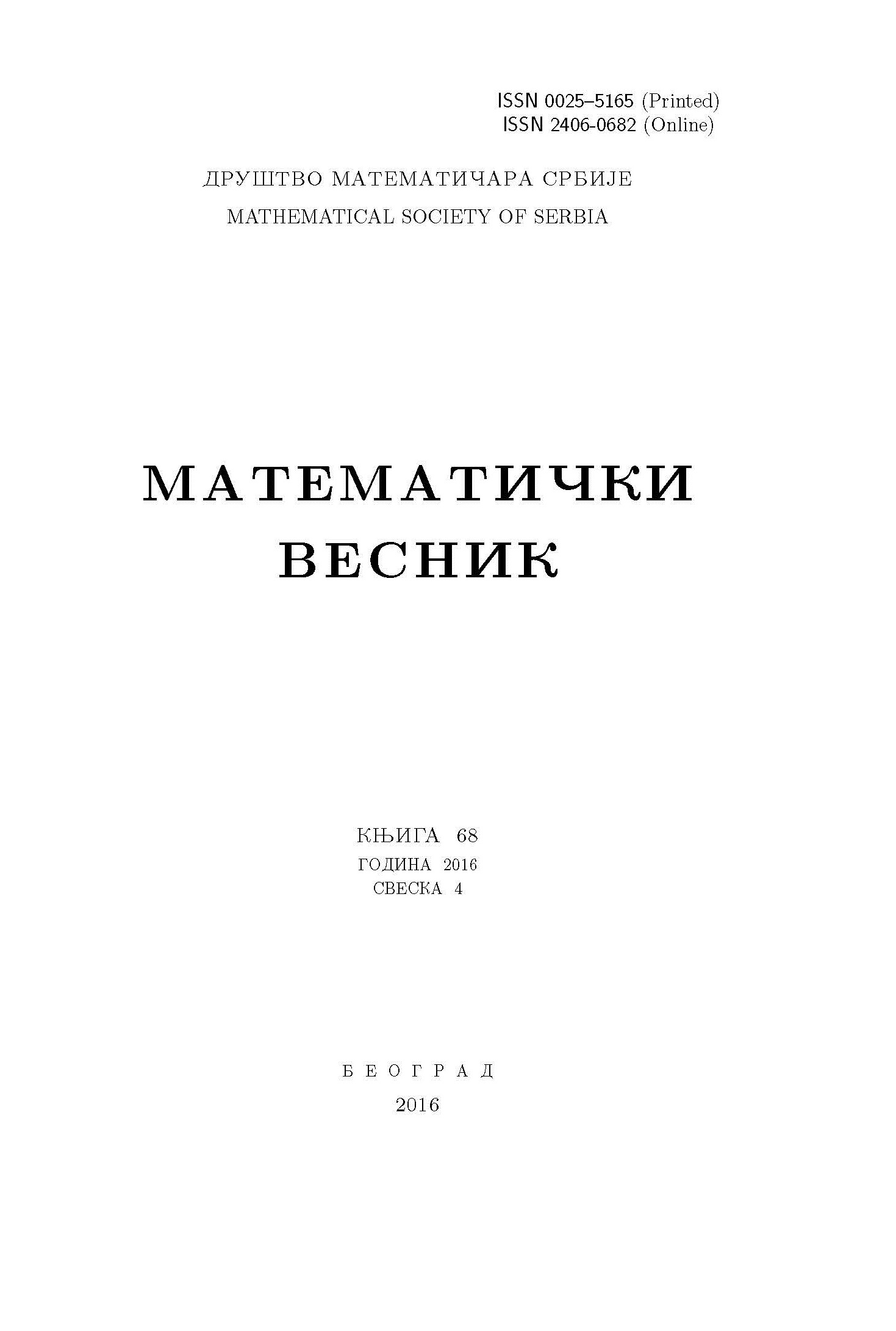﻿ Matematički Vesnik ﻿
 MATEMATIČKI VESNIK МАТЕМАТИЧКИ ВЕСНИКTHREE SOLUTIONS FOR IMPULSIVE FRACTIONAL DIFFERENTIAL EQUATIONS WITH DIRICHLET BOUNDARY CONDITION G. A. Afrouzi, S. Moradi AbstractIn this paper, we discuss the existence of at least three weak solutions for the following impulsive nonlinear fractional boundary value problem \begin{align*} {}_t D_T^{\alpha} \left({}_0^c D_t^{\alpha}u(t)\right) +a(t)u(t)&= \lambda f(t,u(t)), \quad t\neq t_j,\ \text{a.e. } t \in [0, T],\\ \Delta\left({}_t D_T^{\alpha-1} \left({}_0^c D_t^{\alpha}u\right)\right)(t_j)&= I_j(u(t_j)),\quad j=1,\ldots n,\\ u(0) = u(T) &= 0 \end{align*} where $\alpha \in (\frac{1}{2}, 1]$, $a \in C([0, T ])$ and $f : [0, T ]\times\mathbb{R}\to\mathbb{R}$ is an $L^1$-Carathéodory function. Our technical approach is based on variational methods. An example is provided to illustrate the applicability of our results.Keywords: Three solutions; fractional differential equation; impulsive effect; variational methods; critical point theory. MSC: 26A33, 34B15, 35A15, 34B15, 34K45, 58E05 Pages:  1$-$15

﻿